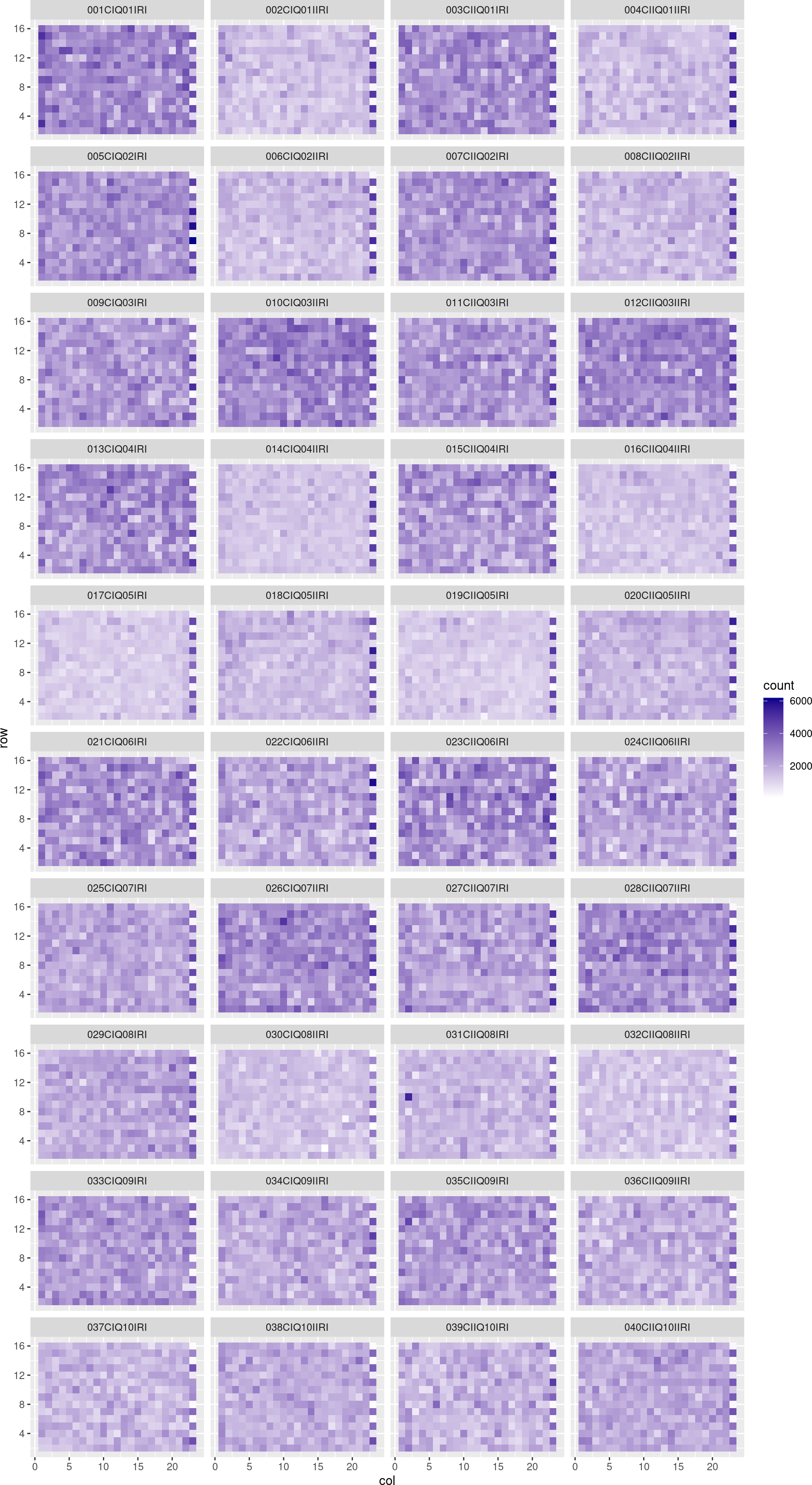# Contents

The `splots` package was written in 2006, and it is still here to support legacy code and to not disrupt other packages that depend on it. It should not be used for new code development. The package provides a single function, `plotScreen`, for visualising data in microtitre plate or slide format. Please consider using the platetools package (see also https://github.com/Swarchal/platetools), or simply ggplot2, as exemplified below.

# 1 Example data

``data("featuresPerWell", package = "HD2013SGI")``

This dataset contains measurements of a combinatorial RNAi screen on a human cell line (HCT116) with fluorescent microscopy as a phenotypic readout, as described in the documentation of the `HD2013SGI` package. There are 168 plates, each with 384 wells. Within each well, the microscope took images at 4 locations, called `field`. Plate, row, column and field ID are given in the following dataframe.

``str(featuresPerWell[])``
``````## 'data.frame':    231840 obs. of  4 variables:
##  \$ plate: chr  "001CIQ01IRI" "001CIQ01IRI" "001CIQ01IRI" "001CIQ01IRI" ...
##  \$ row  : chr  "B" "B" "B" "B" ...
##  \$ col  : chr  "1" "1" "1" "1" ...
##  \$ field: chr  "1" "2" "3" "4" ...``````

The actual measurements are in the following matrix, whose rows are aligned with the above dataframe. Its 353 columns are different morphometric measurements, averaged across the cells in that image.

``str(featuresPerWell[])``
``````##  num [1:231840, 1:353] 2780 3120 2242 2603 2170 ...
##  - attr(*, "dimnames")=List of 2
##   ..\$ : NULL
##   ..\$ : chr [1:353] "count" "nuc.0.m.cx" "nuc.0.m.cy" "nuc.0.m.majoraxis" ...``````

For the purpose of this demo, we only use the first 40 plates and the `count` measurement in field 1, i.e., the number of cells in that field—a proxy for the cells’ viability. We also convert the `row` and `col` variables into integers.

``````library("dplyr")
sgi = tibble(featuresPerWell[], count = featuresPerWell[][, "count"]) |>
filter(plate %in% unique(plate)[1:40],
field == "1") |>
mutate (col = as.integer(col),
row = match(row, LETTERS))
sgi``````
``````## # A tibble: 13,800 × 5
##    plate         row   col field count
##    <chr>       <int> <int> <chr> <dbl>
##  1 001CIQ01IRI     2     1 1      2780
##  2 001CIQ01IRI     2     2 1      2170
##  3 001CIQ01IRI     2     3 1      2548
##  4 001CIQ01IRI     2     4 1      2673
##  5 001CIQ01IRI     2     5 1      2486
##  6 001CIQ01IRI     2     6 1      2611
##  7 001CIQ01IRI     2     7 1      2305
##  8 001CIQ01IRI     2     8 1      2261
##  9 001CIQ01IRI     2     9 1      2724
## 10 001CIQ01IRI     2    10 1      4026
## # ℹ 13,790 more rows``````

# 2 Using `ggplot2`

``````library("ggplot2")
ggplot(sgi, aes(x = col, y = row, fill = count)) + geom_raster() +
facet_wrap(vars(plate), ncol = 4) +
scale_fill_gradient(low = "white", high = "#00008B")``````Figure 1: Visualization using `ggplot`

# 3 Using `splots::plotScreen`

The `plotScreen` takes as input the list `xl`, constructed below. As you can see, this is a lot more clumsy.

``````np = 40
nx = 24
ny = 16
plateNames = unique(featuresPerWell[]\$plate)
assertthat::assert_that(length(plateNames) >= np)
plateNames = plateNames[seq_len(np)]
xl = lapply(plateNames, function(pl) {
sel = with(featuresPerWell[], plate == pl & field == "1")
rv = rep(NA_real_, nx * ny)
r = match(featuresPerWell[]\$row[sel], LETTERS)
c = match(featuresPerWell[]\$col[sel], paste(seq_len(nx)))
i = (r-1) * nx + c
assertthat::assert_that(!any(is.na(r)), !any(is.na(c)), !any(duplicated(i)),
all(r>=1), all(r<=ny), all(c>=1), all(c<=nx))
rv[i] = featuresPerWell[][sel, "count"]
rv
})
names(xl) = plateNames``````

So this the list `xl`:

``length(xl)``
``##  40``
``names(xl)[1:4]``
``##  "001CIQ01IRI"   "002CIQ01IIRI"  "003CIIQ01IRI"  "004CIIQ01IIRI"``
``unique(vapply(xl, function(x) paste(class(x), length(x)), character(1)))``
``##  "numeric 384"``
``xl[][1:30]``
``````##     NA   NA   NA   NA   NA   NA   NA   NA   NA   NA   NA   NA   NA   NA   NA
##    NA   NA   NA   NA   NA   NA   NA   NA   NA 2780 2170 2548 2673 2486 2611``````
``````splots::plotScreen(xl, nx = nx, ny = ny, ncol = 4,
fill = c("white", "#00008B"),
main = "HD2013SGI", legend.label = "count",
zrange = c(0, max(unlist(xl), na.rm = TRUE)))``````
``````## Warning: The function splots::plotScreen is obsolete, please use ggplot with
## geom_raster and facet_wrap instead, as described in the vignette of the splots
## package``````